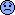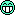# Martha Yeh

Greenhorn
since Jan 31, 2001
Cows and Likes
Cows
0
In last 30 days
0
Total given
0
Likes
0
0
Total given
0
Given in last 30 days
0
Scavenger HuntRanch Hand Scavenger HuntGreenhorn Scavenger Hunt

## Recent posts by Martha YehWhere do I have to put the
/*
<applet code="SampleApplet.class" CodeBase="" width=300 height=400></applet>
*/
In the HTML document?

hello there,
/*
<html>
<applet code="SampleApplet.class" CodeBase="" width=300 height=400></applet>
</html>
*/
save this as "SampleApplet.html"
c:\>javac SampleApplet.java
c:\>appletviewer SampleApplet.htmlmartha
20 years ago
Hello Manfred,
yeah, i know. i tried System.exit(); and stop(); and destory();... man.. its just not work out that way i want.
ok, i guess i need to add the button "exit" or "quit" would be
easier than this??!!thanks very much and good weekendmartha
20 years ago
hello expertspublic void actionPerformed(ActionEvent e){}
I am trying to exit the Applet after type "quit" but not allow me to do that, there must be some wrong...could you help me out?
thanks.
martha
20 years ago
hi there,
yeah, the Unicode characters can appear in the source code.
like:
ch\u0061r a = 'a'; => char a = 'a';
char \u0062 = 'b'; => char b = 'b';
char c = '\u0063'; => char c = 'c';
you can also check Velmurugan Periasamy's webside.. check the FAQ or mocklist from javaranch's website, also back to p.24~25 from Khalid Table 2.7.hope this help. good day.
martha
21 years agohi there..
stevie is right. the ans should be 15, not 20.
"now my question is when the next i=i*5 is executed the value of i should have been 4" .. the operator is excuted the "+" and at the same time excuted "*" or "/".. its like the simple math.
i=(T)(i*(2+ i++))=> excuted the same time, so i=(T)(3*(2+3++))
=>i=15 ..
i didn't increase at all at that time, not separate either.
at the first one: i=i++ + i++ => i=10++ + 11++=> i=21
first i++ ==> 10++, but the next i increase 1 to 11, so i is increase by 1 at next i.
if say, i=i+ i++ => i=10+ 10++ =>i=20, right?!( i didn't increase at all, you can run the simple one and see)also, i remember earlier there were someone discussed this before.. i forgot where it was.. probably a week ago.. you can check it back few days ago. the dicussion was pretty clearhope this help. good day.
martha
21 years ago
it's Trueread p.284 carefully the last 2 pargraph.. u will get itgood weekend again.
martha
21 years ago
the arf[ ] work fine tooforgive me.. sleepy time here.. (12:41am)
goodu got the point.. good weekend again.
martha
21 years ago
hi there..
i tried to run ur code..
***************************
class A0324
{
public static void main(String args[])
{
String s1 = "A";
String s2 = "A";
String s3 = "C";
String s4 = s3;
String s5 = "D";
String s6 = new String("D");
String s7 = new String("E");
String s8 = new String("E");
String s9 = new String("F");
String s10 = "F";
String s11 = new String("H");
String s12 = s11;
/*c1*/ System.out.println("s1 == s2 ==> " + (s1 == s2));//true
/*c2*/ System.out.println("s3 == s4 ==> " + (s3 == s4));//true
/*c3*/ System.out.println("s5 == s6 ==> " + (s5 == s6));//false
/*c4*/ System.out.println("s7 == s8 ==> " + (s7 == s8));//false
/*c5*/ System.out.println("s9 == s10 ==> " +( s9 == s10));//false
/*c6*/ System.out.println("s11 == s12 ==> " + (s11 ==s12));//true
}//EoMain
}//EoA1
************************************
output:
s1==s2==>true
s3==s4==>true
s5==s6==>false
s7==s8==>false
s9==s10==>false
s11==s12==>true
*******************************************
String is immutable.. always remember that.
also, ur code got some error..
* main(String args[ ]) --> not arf[ ]
*System.out.println("s1==s2==>" + (s1==s2));
--> not just "s1==s2==>"+s1==s2
its look like: "s1==s2==>+s1"==s2 // always gets false
hope this help. good weekend.(try it~)
^__^*
martha
21 years ago
hi there,
according the Khalid's book p.284--
If no explicit thread priority is specialed for a thread, it's given the default priority of 5(Thread.NORM_PRIORITY)
also, a thread inherits the priorty of its parent thread. usually the thread scheduler decides to let the thread with the highest priority in the Ready-to-run state to get CPU time.
hope this help. good weekend.^__^*
martha
21 years ago
hi there..
check back ch6.OOP\$6.7Inheritance v.s. Aggregation(P.217)
then you will get it.(Khalid A. Mughal)
good weekend.
martha
21 years ago
Hi all,
yeah, the ans is a,b, and d.
b/c:
a) Flow Layout always honors preferred width and height.
b) Border Layout honors preferred width for its children at EAST and WEST.
d)GridBad Layout honors prefeered width if a component's fill is NONE or VERTICAL.
c)wrong. b/c Grid Layout always dictates width and height, ignoring the components preferred size.
above is according the RHE.
Hope this help.
good day ^__^*
21 years ago
first check those above web sites ^__^* very very good and some simple examples.
here is why:
a=056, so output is 46
b/c: (8^0*6)+(8^1*5)=(1*6)+(8*5)=6+40=46
b=034, so output is 28
b/c: (8^0*4)+(8^1*3)=(1*4)+(8*3)=4+24=28
hope this help ^__^*
good day.
21 years ago
here is the hierachy of the collection:
Collection-->List
Collection-->Set--> SortedSet
Map-->SortedMap
also, the Collection interfacces and the classes that implement them:
Set-->HashSet
SortedSet-->TreeSet
Map-->HashMap, HashTable
SortedMap-->TreeMap
hope this will help.
good day ^__^*
21 years ago
mm.. i cc.. thanks a lot ^__^*
21 years ago
Hi all,
this question is from:http://www.jchq.net/tutorial/05_04Tut.htm
class ValHold{
public int i=10;
}
public class ObParm{
public static void main(String argv[]){
ObParm o=new ObParm();
o.amethod();
}
public void amethod(){
int i=99;
ValHold v=new ValHold();
v.i=30;
another(v,i);
System.out.println(v.i);
}//end of amethod
public void another(ValHold v, int i){
i=0;
v.i=20;
ValHold vh=new ValHold();
v=vh;
System.out.println(v.i+" "+i);
}//end of another
}
what's output:
1)10,0,30
2)20,0,30
3)20,99,30
4)10,0,20
output is 10,0
20
my question is: b/c another method passed the field of the object, and v=vh, so the output is 10 (b/c v.i=10 from class ValHold), and i=0 (b/c another method i=0) mmm.. but, why in amethod "System.out.println(v.i);" print out v.i=20?
b/c another method's v.i=20? or say vh.i=20?...???..
please explain why, why, why... ^__^*
thanks a lot.
martha
p.s. ohh.. ans. 4) and good day.
21 years ago